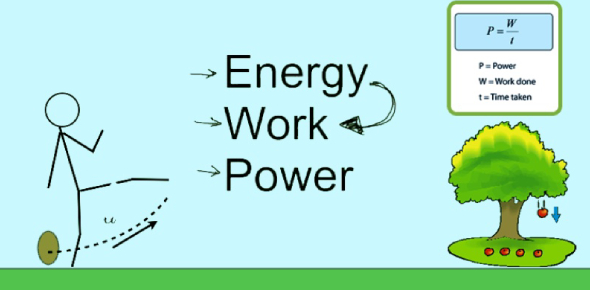# Work And Energy Questions! Science Trivia Quiz

15 Questions | Attempts: 2490SettingsWhat do you know about work and energy? Do you think you know as much as necessary to pass this quiz? Work done is generally referred in relation to the force applied while energy is used about other factors such as heat. There is a powerful connection between work and energy. Take this quiz and test your knowledge of work and energy.

• 1.
A man is carrying the heavy luggage from one platform to the other of a Railway station but still according to the logic of science his work done is said to be zero. Can you opt-out of the correct reason?
• A.

The force is acting along the direction of displacement of luggage

• B.

The force is acting perpendicular to the direction of displacement of luggage

• C.

The force is acting opposite to the direction of displacement of luggage

• D.

None of these

• 2.
When a body like the earth is moving in a circular path the work done in that case is zero because:
• A.

Centripetal force acts in the direction of motion of the body

• B.

Centripetal force acts along the radius of circular path

• C.

Gravitational force acts along the radius of circular path

• D.

Centrifugal force acts perpendicular to the radius of circular path

• 3.
A car is accelerated on a leveled road and attains a velocity 4 times of its initial velocity. In this process, the kinetic energy of the car.
• A.

Becomes twice to that of the initial

• B.

Becomes four times to that of the initial

• C.

Remains the same

• D.

Becomes 16 times to that of the initial

• 4.
The momentum of a bullet of mass 20 g fired from a gun is 10 kg.m/s. The kinetic energy of this bullet in kJ will be:
• A.

25

• B.

2.5

• C.

0.25

• D.

5

• 5.
You would have observed a runner who possesses only kinetic energy due to his motion. Can you guess which form of energy does a flying bird have?
• A.

Kinetic energy

• B.

Potential energy

• C.

Both kinetic and potential energy

• D.

Can’t say

• 6.
Which one of the following is not the unit of energy?
• A.

Kilowatt

• B.

Kilowatt hour

• C.

Joule

• D.

Newton meter

• 7.
In the dams, water is stored in the high reservoirs and then made to fall down. This falling water then rotates the turbines to generate electricity. In this energy conversion process can you tell the initial and final energies respectively?
• A.

Kinetic energy and electrical energy

• B.

Potential energy and kinetic energy

• C.

Potential energy and electrical energy

• D.

Kinetic energy and potential energy

• 8.
A man of mass 50 kg jumps to a height of 1 m. His potential energy at the highest point is (g = 10 m/s2):
• A.

50 J

• B.

500 J

• C.

5 J

• D.

5000 J

• 9.
The type of energy possessed by a simple pendulum, when it is at the mean position is:
• A.

Kinetic energy

• B.

Potential energy

• C.

Kinetic + Potential energy

• D.

Sound energy

• 10.
A body is falling from a height h. After it has fallen to a height of h/2, it will possess:
• A.

Only kinetic energy

• B.

Half kinetic and half potential energy

• C.

Only potential energy

• D.

More kinetic and less potential energy

• 11.
Two objects of masses 1 x 10-3 kg and 4 x 10-3 kg have equal momentum. What is the ratio of their kinetic energies?
• A.

2:1

• B.

4:1

• C.

1:4

• D.

1:2

• 12.
The work done on an object does not depend upon the:
• A.

Angle between force and displacement

• B.

Force applied

• C.

Initial velocity of the object

• D.

Displacement

• 13.
An iron sphere of mass 10 kg has the same diameter as an aluminum sphere of mass 3.5 kg. Both spheres are dropped simultaneously from a tower. When they are 10 m above the ground, they have the same:
• A.

Potential energy

• B.

Momenta

• C.

Acceleration

• D.

Kinetic energy

• 14.
A microphone converts:
• A.

Sound energy into mechanical energy in stereo system.

• B.

Sound energy into electrical energy in public address system.

• C.

Electrical energy into sound energy in ordinary telephone.

• D.

Microwave energy into sound energy in a mobile phone.

• 15.
Which of the following energy change involves frictional force? a) Potential energy to sound energy b) Chemical energy to heat energy c) Kinetic energy to heat energy d) Chemical energy to heat energy Choose the correct option among the following:
• A.

Both a and b

• B.

Only d

• C.

Both b and c

• D.

Only c

## Related TopicsBack to top
×

Wait!
Here's an interesting quiz for you.